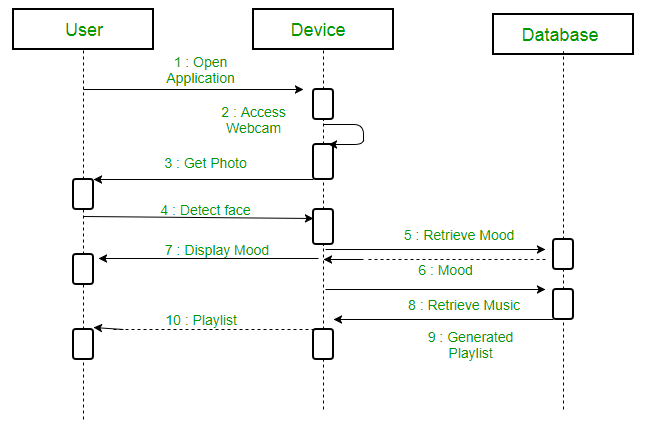Related Articles

# Difference between Sequence Diagram and Activity Diagram

• Last Updated : 06 Mar, 2019

What is Sequence Diagram ?

A sequence diagram simply depicts interaction between objects in a sequential order i.e. the order in which these interactions take place. We can also use the terms event diagrams or event scenarios to refer to a sequence diagram. Sequence diagrams describe how and in what order the objects in a system function. These diagrams are widely used by businessmen and software developers to document and understand requirements for new and existing systems.

Example: A sequence diagram for an emotion based music player:What is Activity Diagram ?

An Activity Diagram is basically a flowchart (Unified Modelling Language) diagram which is used to describe the dynamic aspect of the system. the flowchart represents the flow of activities from one activity to another activity. The activities can be described as the operation of a System. The flow of control in the activity diagram is drawn from one operation to another. This flow can be sequential, branched or concurrent.

Example: An activity diagram for an emotion based music playerSimilarities Between Sequence and Activity Diagram:

• Both Sequence Diagram and Activity Diagram are UML diagrams.
• Both Sequence and Activity Diagrams are used to represent the control flow of messages.

Differences Between Sequence Diagram and Activity Diagram

Sequence DiagramActivity Diagram
The Sequence diagram represents the UML, which is used to visualize the sequence of calls in a system that is used to perform a specific functionality.The Activity diagram represents the UML, which is used to model the workflow of a system.
The Sequence diagram shows the message flow from one object to another object.The Activity diagram shows the message flow from one activity to another.
Sequence diagram is used for the purpose of dynamic modelling.Activity diagram is used for the purpose of functional modelling.
Sequence diagram is used to describe the behavior of several objects
in a single use case
Activity diagrams is used to describe the general sequence
of actions for several objects and use cases.
Sequence diagram is mainly used to represent the time order of a process.Activity diagram is used to represent the execution of the process.
My Personal Notes arrow_drop_up